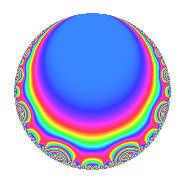# Properties

 Label 4008.2.a.eLevel 4008 Weight 2 Character orbit 4008.a Self dual yes Analytic conductor 32.004 Analytic rank 1 Dimension 3 CM no Inner twists 1

# Related objects

## Newspace parameters

 Level: $$N$$ = $$4008 = 2^{3} \cdot 3 \cdot 167$$ Weight: $$k$$ = $$2$$ Character orbit: $$[\chi]$$ = 4008.a (trivial)

## Newform invariants

 Self dual: yes Analytic conductor: $$32.0040411301$$ Analytic rank: $$1$$ Dimension: $$3$$ Coefficient field: 3.3.148.1 Coefficient ring: $$\Z[a_1, \ldots, a_{5}]$$ Coefficient ring index: $$1$$ Twist minimal: yes Fricke sign: $$1$$ Sato-Tate group: $\mathrm{SU}(2)$

## $q$-expansion

Coefficients of the $$q$$-expansion are expressed in terms of a basis $$1,\beta_1,\beta_2$$ for the coefficient ring described below. We also show the integral $$q$$-expansion of the trace form.

 $$f(q)$$ $$=$$ $$q - q^{3} + ( 2 - \beta_{2} ) q^{5} + ( -2 + 2 \beta_{1} ) q^{7} + q^{9} +O(q^{10})$$ $$q - q^{3} + ( 2 - \beta_{2} ) q^{5} + ( -2 + 2 \beta_{1} ) q^{7} + q^{9} + ( -1 - \beta_{1} + \beta_{2} ) q^{11} + 2 q^{13} + ( -2 + \beta_{2} ) q^{15} + 3 \beta_{2} q^{17} + ( 2 - 2 \beta_{1} - 2 \beta_{2} ) q^{19} + ( 2 - 2 \beta_{1} ) q^{21} -8 q^{23} + ( 2 - \beta_{1} - 5 \beta_{2} ) q^{25} - q^{27} + ( -4 - 2 \beta_{1} + 2 \beta_{2} ) q^{29} + ( -2 + 2 \beta_{1} + 2 \beta_{2} ) q^{31} + ( 1 + \beta_{1} - \beta_{2} ) q^{33} + ( -2 + 2 \beta_{1} + 2 \beta_{2} ) q^{35} + ( -4 + 2 \beta_{1} ) q^{37} -2 q^{39} + ( 6 - 6 \beta_{1} - 3 \beta_{2} ) q^{41} + ( -2 - 2 \beta_{1} - 3 \beta_{2} ) q^{43} + ( 2 - \beta_{2} ) q^{45} + ( 1 - 3 \beta_{1} + 3 \beta_{2} ) q^{47} + ( 5 - 4 \beta_{1} + 4 \beta_{2} ) q^{49} -3 \beta_{2} q^{51} + ( -4 + 4 \beta_{1} + 3 \beta_{2} ) q^{53} + ( -6 + 4 \beta_{2} ) q^{55} + ( -2 + 2 \beta_{1} + 2 \beta_{2} ) q^{57} + ( 2 - 2 \beta_{1} + 2 \beta_{2} ) q^{59} + ( -1 + 5 \beta_{1} + 5 \beta_{2} ) q^{61} + ( -2 + 2 \beta_{1} ) q^{63} + ( 4 - 2 \beta_{2} ) q^{65} + ( -8 - 2 \beta_{1} - \beta_{2} ) q^{67} + 8 q^{69} + ( -4 - 4 \beta_{1} - 6 \beta_{2} ) q^{71} + ( 8 - 2 \beta_{1} - 4 \beta_{2} ) q^{73} + ( -2 + \beta_{1} + 5 \beta_{2} ) q^{75} + ( -4 - 4 \beta_{2} ) q^{77} + ( 4 - 2 \beta_{1} + 5 \beta_{2} ) q^{79} + q^{81} + ( -4 + 8 \beta_{1} + 6 \beta_{2} ) q^{83} + ( -9 + 3 \beta_{1} + 9 \beta_{2} ) q^{85} + ( 4 + 2 \beta_{1} - 2 \beta_{2} ) q^{87} + ( -6 \beta_{1} + 2 \beta_{2} ) q^{89} + ( -4 + 4 \beta_{1} ) q^{91} + ( 2 - 2 \beta_{1} - 2 \beta_{2} ) q^{93} + ( 8 - 4 \beta_{1} - 8 \beta_{2} ) q^{95} + ( 2 - 6 \beta_{2} ) q^{97} + ( -1 - \beta_{1} + \beta_{2} ) q^{99} +O(q^{100})$$ $$\operatorname{Tr}(f)(q)$$ $$=$$ $$3q - 3q^{3} + 6q^{5} - 4q^{7} + 3q^{9} + O(q^{10})$$ $$3q - 3q^{3} + 6q^{5} - 4q^{7} + 3q^{9} - 4q^{11} + 6q^{13} - 6q^{15} + 4q^{19} + 4q^{21} - 24q^{23} + 5q^{25} - 3q^{27} - 14q^{29} - 4q^{31} + 4q^{33} - 4q^{35} - 10q^{37} - 6q^{39} + 12q^{41} - 8q^{43} + 6q^{45} + 11q^{49} - 8q^{53} - 18q^{55} - 4q^{57} + 4q^{59} + 2q^{61} - 4q^{63} + 12q^{65} - 26q^{67} + 24q^{69} - 16q^{71} + 22q^{73} - 5q^{75} - 12q^{77} + 10q^{79} + 3q^{81} - 4q^{83} - 24q^{85} + 14q^{87} - 6q^{89} - 8q^{91} + 4q^{93} + 20q^{95} + 6q^{97} - 4q^{99} + O(q^{100})$$

Basis of coefficient ring in terms of a root $$\nu$$ of $$x^{3} - x^{2} - 3 x + 1$$:

 $$\beta_{0}$$ $$=$$ $$1$$ $$\beta_{1}$$ $$=$$ $$\nu$$ $$\beta_{2}$$ $$=$$ $$\nu^{2} - \nu - 2$$
 $$1$$ $$=$$ $$\beta_0$$ $$\nu$$ $$=$$ $$\beta_{1}$$ $$\nu^{2}$$ $$=$$ $$\beta_{2} + \beta_{1} + 2$$

## Embeddings

For each embedding $$\iota_m$$ of the coefficient field, the values $$\iota_m(a_n)$$ are shown below.

For more information on an embedded modular form you can click on its label.

Label $$\iota_m(\nu)$$ $$a_{2}$$ $$a_{3}$$ $$a_{4}$$ $$a_{5}$$ $$a_{6}$$ $$a_{7}$$ $$a_{8}$$ $$a_{9}$$ $$a_{10}$$
1.1
 −1.48119 2.17009 0.311108
0 −1.00000 0 0.324869 0 −4.96239 0 1.00000 0
1.2 0 −1.00000 0 1.46081 0 2.34017 0 1.00000 0
1.3 0 −1.00000 0 4.21432 0 −1.37778 0 1.00000 0
 $$n$$: e.g. 2-40 or 990-1000 Significant digits: Format: Complex embeddings Normalized embeddings Satake parameters Satake angles

## Inner twists

This newform does not admit any (nontrivial) inner twists.

## Twists

By twisting character orbit
Char Parity Ord Mult Type Twist Min Dim
1.a even 1 1 trivial 4008.2.a.e 3
4.b odd 2 1 8016.2.a.n 3

By twisted newform orbit
Twist Min Dim Char Parity Ord Mult Type
4008.2.a.e 3 1.a even 1 1 trivial
8016.2.a.n 3 4.b odd 2 1

## Atkin-Lehner signs

$$p$$ Sign
$$2$$ $$-1$$
$$3$$ $$1$$
$$167$$ $$-1$$

## Hecke kernels

This newform subspace can be constructed as the intersection of the kernels of the following linear operators acting on $$S_{2}^{\mathrm{new}}(\Gamma_0(4008))$$:

 $$T_{5}^{3} - 6 T_{5}^{2} + 8 T_{5} - 2$$ $$T_{7}^{3} + 4 T_{7}^{2} - 8 T_{7} - 16$$